## Texture Synthesis Using Convolutional Neural Networks

2015a, Gatys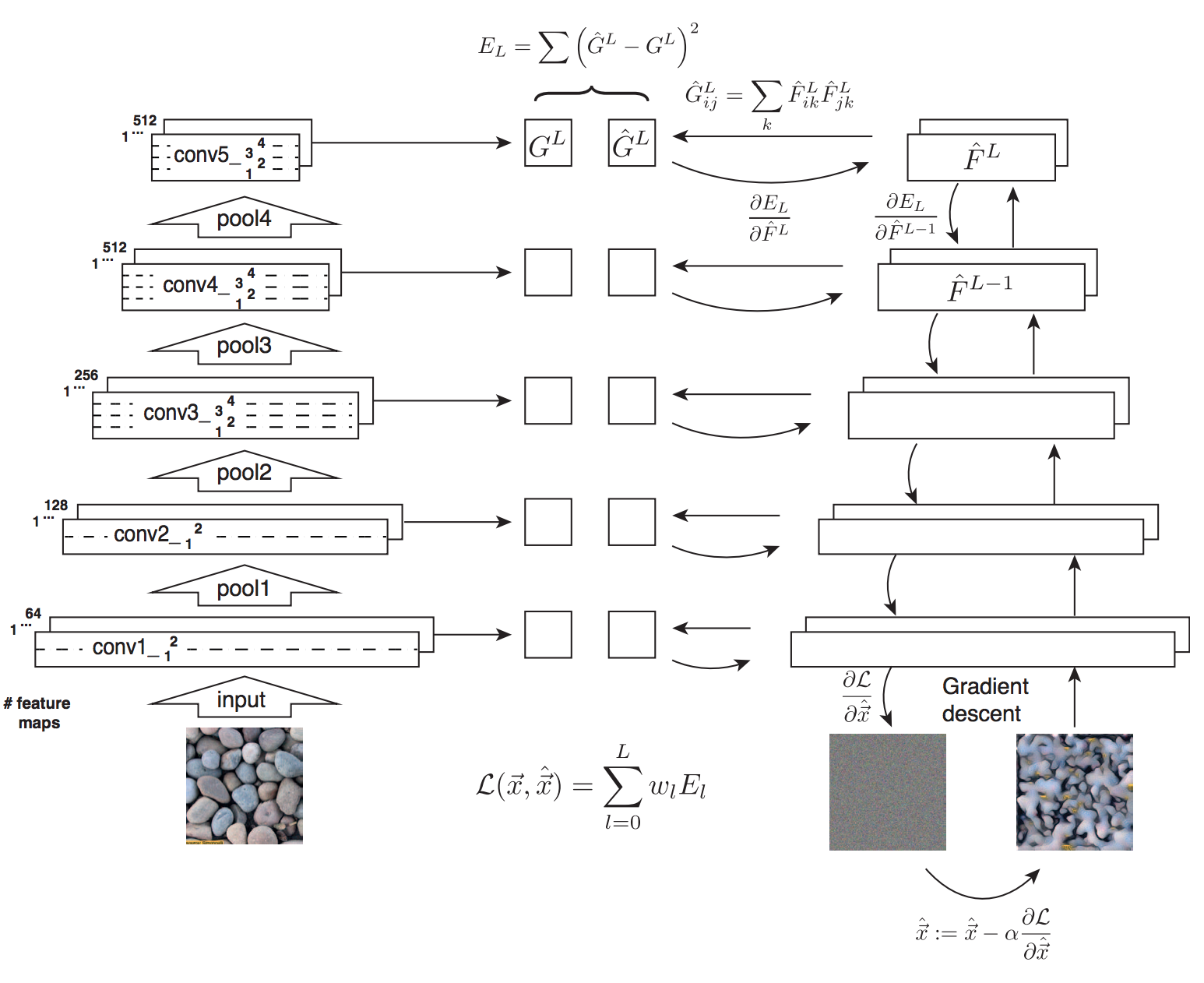1. 将图片输入预训练好的神经网络(VGG-19)中， 生成每层的特征
2. 将灰度图同样输入预训练好的神经网络中，生成每层的特征
3. 计算每层特征的Gram矩阵，计算两张图片对应层的Gram矩阵的平方误差，再将每层的误差按权求和，获得损失函数
4. 计算损失函数对灰度图每个像素的梯度，进行梯度下降，最终得到输出图。这里进行梯度下降的不是模型参数，而是输入像素。这也是Deep Dream的主要思路。

$$E_{l}(S, \hat{X}) = \frac{1}{4C_{in}^2C_{out}^2}\sum\limits_{i,j}(G_{ij} - \hat{G}_{ij})^2$$

$$\mathcal{L}_{style}(S, \hat{X}) = \sum\limits_{l}w_{l}E_{l}$$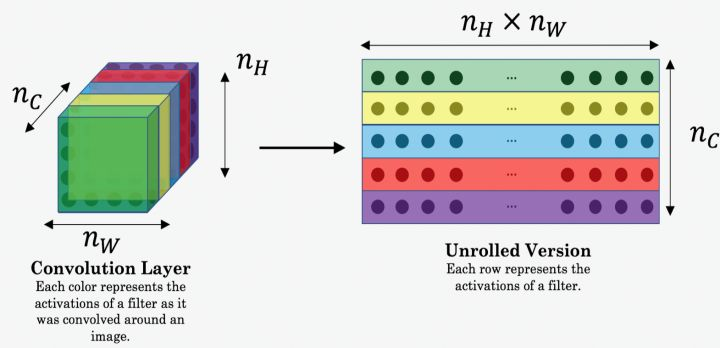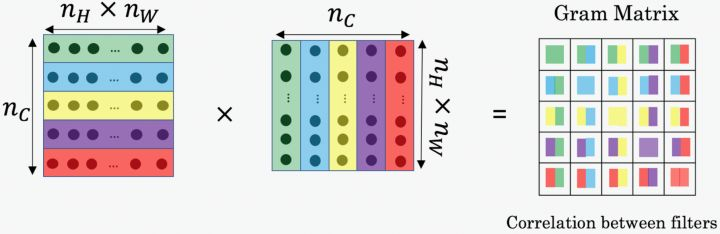## A Neural Algorithm of Artistic Style

2015b, Gatys

$$\mathcal{L}_{content}(P, \hat{X}) = \frac{1}{2}\sum\limits_{i,j}(F_{ij} - \hat{F}_{ij})^2$$

$$\mathcal{L}(P, S, \hat{X}) = \alpha\mathcal{L}_{content}(P, \hat{X}) + \beta\mathcal{L}_{style}(S, \hat{X})$$

## Perceptual Losses for Real-Time Style Transfer and Super-Resolution

Mar 2016, Justin Johnson

### Feature Reconstruction Loss

$$\mathcal{L^{j}_{content}}(y, \hat{y}) = \frac{1}{C_{j}H_{j}W_{j}} \Vert \phi_{j}(y) - \phi_{j}(\hat{y}) \Vert^{2}_{2}$$

### Style Reconstruction Loss

$$\mathcal{L^{j}_{style}}(y, \hat{y}) = \frac{1}{C_{j}H_{j}W_{j}}\Vert G^{\phi}_{j}(y) - G^{\phi}_{j}({\hat{y}}) \Vert^{2}_{F}$$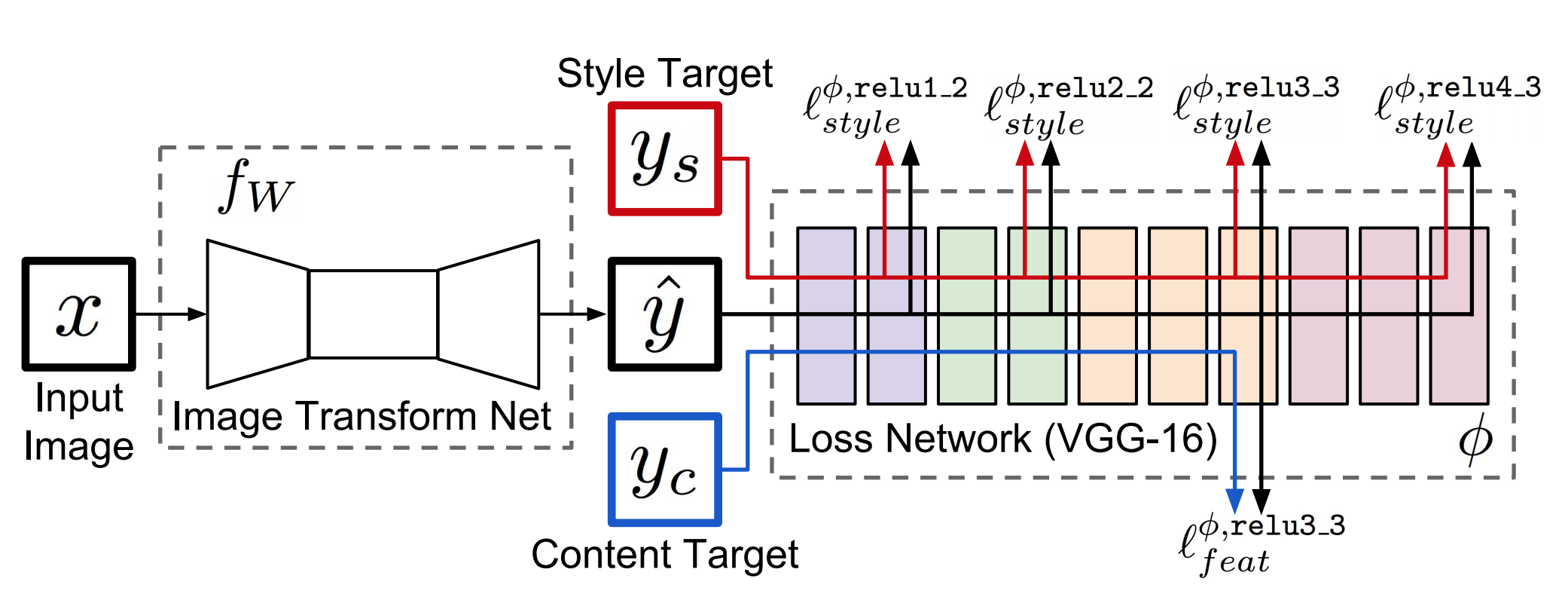## Fast Patch-based Style Transfer of Arbitrary Style

Dec 2016, Tian Qi Chen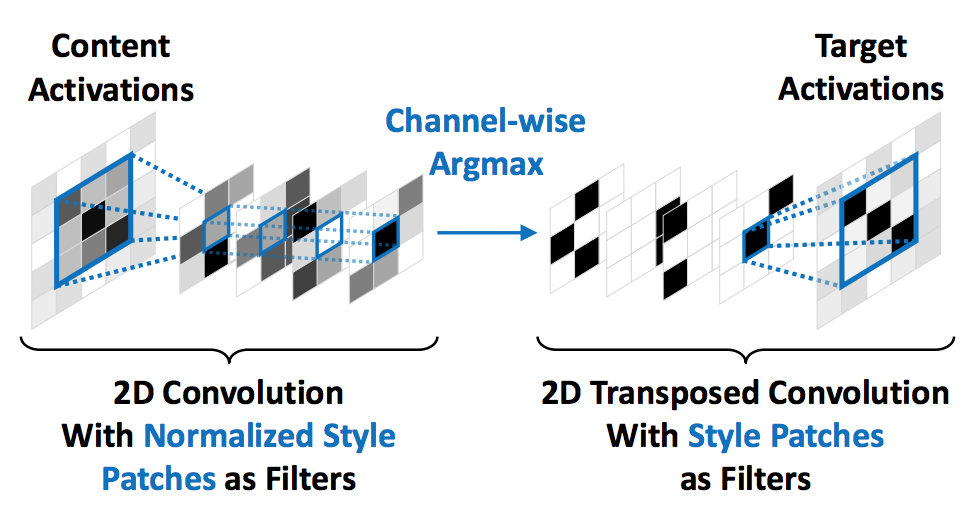### Style Swap

1. Content图片产生特征图$\phi(C)$
2. Style图片产生特征图$\phi(S)$
3. 用$\phi(S)$做卷积核扫过$\phi(C)$,寻找最相似的